Latest Banking jobs   »

# Quantitative Aptitude Quiz For IBPS RRB PO Prelims 2022- 9th June

Q1. Neha invested an amount of Rs.6400 at the rate of R% p.a. on compound interest. If she gets a total amount of Rs. 1700 as interest at the end of 2 years, then find R%?
(a) 12.50%
(b) 25%
(c) 20%
(d) 17.50%
(e) 10%

Q2. P and Q start a business jointly. P invests Rs. 21000 for 9 months and Q invest Rs. X for 3 months. If Q gets two-third of total profit, then find the amount invested by Q. (in Rupees.)
(a) 63000
(b) 84000
(c) 42000
(d) 123000
(e) 126000

Q3. The ratio of cost price and market price of an article is 24:27. If 10% discount is given for article, then find the profit percentage?
(a) 1.25%
(b) 1.75%
(c) 1.50%
(d) 2.50%
(e) 2.25%

Q4. P, Q, R together invested Rs. 100000 in a business. if P invested Rs. 8000 more than Q and Q invested Rs. 10000 more than R, then at the end of the year what will be share of P out of a total profit of Rs. 70,000?
(a) 30600 Rs.
(b) 28000 Rs.
(c) 27500 Rs.
(d) 29400 Rs.
(e) 30000 Rs.

Q5. A merchant has 5000 kg of rice, some part of which he sells at 12 ½ % profit and the rest part he sells at 25% profit. He gains 22 ½ % on the whole. Find the Quantity that he sold at 12 ½ % profit?
(a) 1250 kg
(b) 1000 kg
(c) 1500 kg
(d) 2000 kg
(e) 1100 kg

Q6. What will be amount on Rs. 64000 in one and half years if it is invested at 15% p.a. compound interest, interest being compounded half-yearly?
(a) 85000 Rs.
(b) 82597 Rs
(c) 79507 Rs
(d) 77658 Rs
(e) 75987 Rs.

Q7. The simple interest on a capital is 49/400 of the capital. If both are numerically equal, then find the rate percent and time?
(a) 3.5%, 3.5 years
(b) 4.0%, 4.0 years
(c) 3.0%, 3.0 years
(d) 2.8%, 2.8 years
(e) 2.5%, 2.5 years

Q8. Mohan and Ravi started a business by investing Rs75000 and Rs45,000 respectively. After 8 Months Keshav Joined by investing Rs. X. If at the end of the year profit earned by Keshav is equal to average of profits earned by Mohan and Ravi together then find the value of X?
(a) 180000 Rs.
(b) 190000 Rs.
(c) 170000 Rs.
(d) 160000 Rs.
(e) 150000 Rs.

Q9. For what sum the simple interest at R/4% p.a. for 3 years will be Rs. 12/5 R? (in rupees)
(a) 250
(b) 300
(c) 320
(d) 360
(e) 420

Q10. A book is marked 80% above cost price and sold at 40% discount, if difference between discount given and profit earned is Rs.460.8, then find M.R.P. of book?
(a) Rs.720
(b) Rs.1200
(c) Rs.1296
(d) Rs. 777.60
(e) Rs.1660

Q11. Raj and Rahul invested in a business and investment of Raj is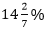more than that of Rahul. If Raj withdrew his investment after 7 months and they got a total profit of Rs. 6000 at the end of the year. then find the profit share of Raj is how much less or more than that of Rahul?
(a) Rs 1400
(b) Rs 1180
(c) Rs 1150
(d) Rs 1400
(e) Rs 1200

Q12. Compound interest on a certain sum at the rate of 20% payable half yearly for 1.5 years is Rs.’77.5’ more than the Simple interest for 1.5 years on same sum at the same rate (p.a.). Find the Sum?
(a) Rs. 2600
(b) Rs. 2200
(c) Rs. 2700
(d) Rs. 2300
(e) Rs. 2500

Q13. A shopkeeper marked up price of an article by x% above its cost price and offers a discount of (x-20) % on it, so that the selling price becomes Rs. 24 more than its cost price. If ratio of cost price to profit is of 25:3, then find the value of ‘x’.
(a) 25
(b) 40
(c) 20
(d) 50
(e) 100

Q14. A and B start a business with Rs. 1750 and Rs. 5250 respectively. After 4 months C joins the business with Rs. 3500 and B withdraw his 50% investment. At the end of the year, B gets Rs.3000 as his share of profit, then find the profit share of C is how much more than that of A in the business?
(a) Rs. 600
(b) Rs. 500
(c) Rs. 700
(d) Rs. 400
(e) Rs. 800

Q15. A shopkeeper sold a jeans at 20% profit and a shirt at a profit of 25%. If the cost price of the shirt is equal to the selling price of jeans and shopkeeper earned an overall profit of Rs. 400, then find the sum of selling price of both the articles together?
(a) 2160 Rs.
(b) 1760 Rs.
(c) 1960 Rs.
(d) 2260 Rs.
(e) 2460 Rs.

Solutions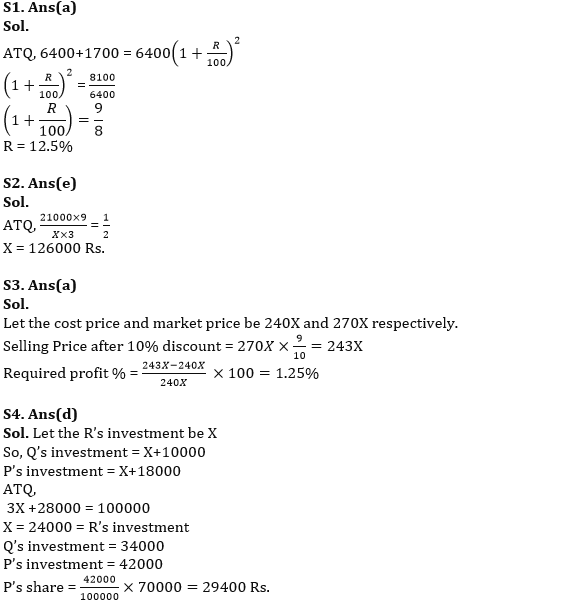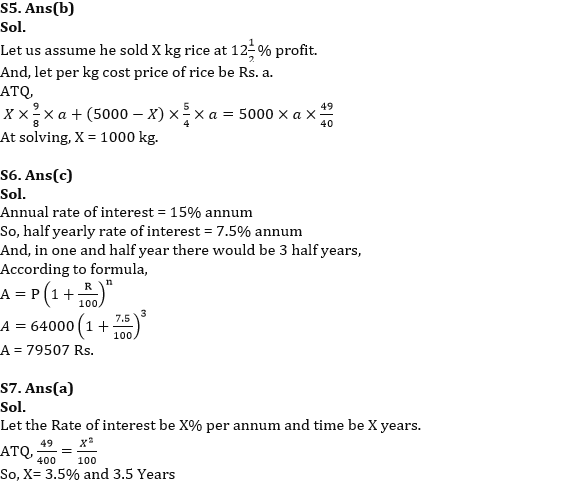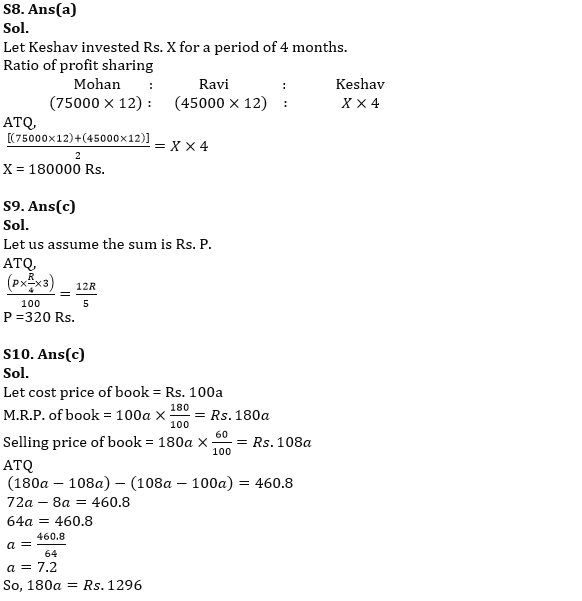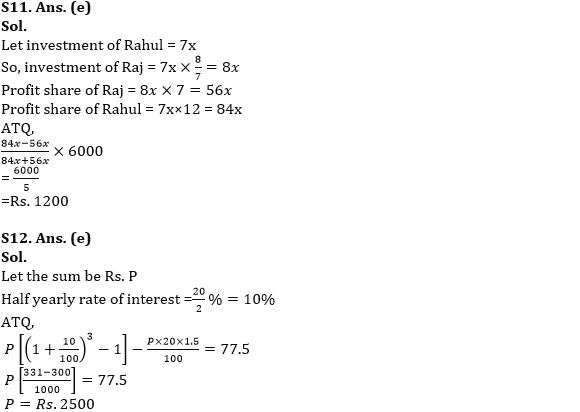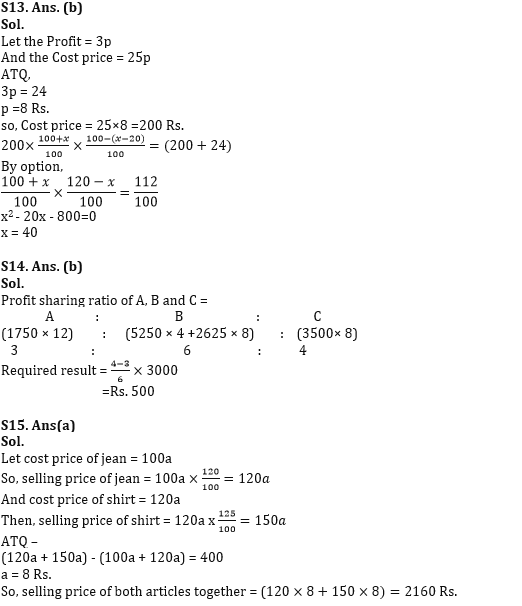#### Congratulations!